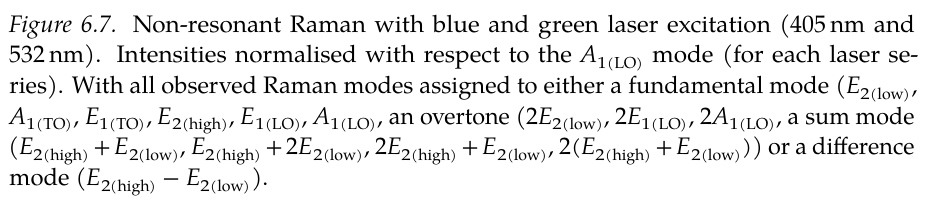Have you also been in the situation where you need to write up your latest Raman spectra of ZnO?
And just exasperated at the thought of typing up all those mode assignments using LaTeX math notation (because honestly, it looks good).

I created this macro, \nonresmode{E2h}[E2l][long][diff], to make it easier to write.
Now all I have to do is write, for example, \nonresmode{A1LO}, and LaTeX does all the work and produces a nicely typeset label.

Here is some example LaTeX source:

Non-resonant Raman with green laser excitation (\qty{532}{\nm}).
All the observed Raman modes could be assigned to either a fundamental mode
(\nonresmode{E2l}[][long], \nonresmode{A1TO}, \nonresmode{E1TO}, \nonresmode{E2h}[][long],
\nonresmode{E1LO}, \nonresmode{A1LO}),  an overtone (\nonresmode{2E2l}[][long], \nonresmode{2E1LO},
\nonresmode{2A1LO}), a sum mode (\nonresmode{E2h}[E2l][long], \nonresmode{E2h}[2E2l][long],
\nonresmode{2E2h}[E2l][long], $2(\nonresmode{E2h}[E2l][long])$) or
a difference mode (\nonresmode{E2h}[E2l][long][diff]).

and the resulting output:And here is the macro definitions (to avoid repeating the main "correspondence table" between the short-codes and the typeset text,
I opted to define multiple functions. I also made use of the beautiful macro by egreg that defines a case-like environment:

% defines a case environment
% code taken from a TeX.SE answer by egreg
% https://tex.stackexchange.com/a/451094/10824
% fantastic piece of code, works beautifully, for an arbitrary number of cases
\ExplSyntaxOn
\NewExpandableDocumentCommand{\stringcase}{mO{}m}{%
\str_case_e:nnF { #1 } { #3 } { #2 }
}
\ExplSyntaxOff

% not meant to be exposed to user
\NewDocumentCommand{\NonResonantModesShort}{m}{%
\stringcase{#1}[\textbf{??}]{%
% note, \text{} inside math environment will obey font settings from
% surrounding environment (e.g., bold) but \mathrm{} will not which
% makes it better for this purpose
% {shortcode}{LaTeX typeset text}
{E2l}{\ensuremath{E_{2\mathrm{l}}}}%
{A1TO}{\ensuremath{A_{1(\mathrm{TO})}}}%
{E1TO}{\ensuremath{E_{1(\mathrm{TO})}}}%
{E2h}{\ensuremath{E_{2\mathrm{h}}}}%
{E1LO}{\ensuremath{E_{1(\mathrm{LO})}}}%
{A1LO}{\ensuremath{A_{1(\mathrm{LO})}}}%
{2E2l}{\ensuremath{2E_{2\mathrm{l}}}}%
{2E2h}{\ensuremath{2E_{2\mathrm{h}}}}%
{2E1LO}{\ensuremath{2E_{1(\mathrm{LO})}}}%
{2A1LO}{\ensuremath{2A_{1(\mathrm{LO})}}}%
}%
}

% not meant to be exposed to user
\NewDocumentCommand{\NonResonantModesLong}{m}{%
\stringcase{#1}[\textbf{??}]{%
{E2l}{\ensuremath{E_{2\mathrm{(low)}}}}%
{A1TO}{\ensuremath{A_{1(\mathrm{TO})}}}%
{E1TO}{\ensuremath{E_{1(\mathrm{TO})}}}%
{E2h}{\ensuremath{E_{2\mathrm{(high)}}}}%
{E1LO}{\ensuremath{E_{1(\mathrm{LO})}}}%
{A1LO}{\ensuremath{A_{1(\mathrm{LO})}}}%
{2E2l}{\ensuremath{2E_{2\mathrm{(low)}}}}%
{2E2h}{\ensuremath{2E_{2\mathrm{(high)}}}}%
{2E1LO}{\ensuremath{2E_{1(\mathrm{LO})}}}%
{2A1LO}{\ensuremath{2A_{1(\mathrm{LO})}}}%
}%
}

% not meant to be exposed to user
\NewDocumentCommand{\NonResonantModesLogic}{m O{short}}{%
\IfNoValueTF{#2}{%
% arg #2 (optional) not given, proceed as "short"
\NonResonantModesShort{#1}%
}{%
% arg #2 (optional) was given, check if it is "long"
\ifthenelse{\equal{#2}{long}}{%
% "long" was given
\NonResonantModesLong{#1}%
}{%
% arg was given, but is not equal to "long"
% check if it is "short"
\ifthenelse{\equal{#2}{short}}{%
\NonResonantModesShort{#1}%
}{%
% arg was neither "long" nor "short", something else
\NonResonantModesShort{}%
}%
}%
}%
}

% Non-resonant Raman modes of ZnO
% \nonresmode{E2h}[E2l][long][diff]
% Use \nonresmode like this:
% \nonresmode{E2l}              => E2l
% \nonresmode{E2l}[][long]      => E2low
% \nonresmode{}                 => ??
% \nonresmode                   => ERROR
% \nonresmode{E2l}[E2h]         => E2l+E2h
% \nonresmode{E2l}[E2h][][diff] => E2l-E2h
% \nonresmode{E2l}[E2h][long]   => E2low + E2high
% only specify optional arguments if necessary, i.e.,
% remember, optional arg "o" will supply the special -NoValue- marker if not given
% the arg O{sum} is an optional arg with the default value "sum"
\NewDocumentCommand{\nonresmode}{m o O{short} O{sum}}{%
\IfNoValueTF{#2}{%
% no arg #2 was given, which means we are typesetting a single mode
% if no arg #2 was given, then we can disregard #4
\IfNoValueTF{#3}{%
% this way, if #3 was not given, we avoid passing the special -NoValue- marker
\NonResonantModesLogic{#1}%
}{%
% if #3 was given, pass it on, unless it was empty
\ifthenelse{\isempty{#3}}{%
\NonResonantModesLogic{#1}%
}{%
\NonResonantModesLogic{#1}[#3]%
}%
}%
}{%
% If optional arg #2 was given and is empty, perform the same code as if -NoValue-
\ifthenelse{\isempty{#2}}{%
\IfNoValueTF{#3}{%
% this way, if #3 was not given, we avoid passing the special -NoValue- marker
\NonResonantModesLogic{#1}%
}{%
% if #3 was given, pass it on, unless it was empty
\ifthenelse{\isempty{#3}}{%
\NonResonantModesLogic{#1}%
}{%
\NonResonantModesLogic{#1}[#3]%
}%
}%
}{%
% if #2 is not -NoValue- nor empty, then just assume that it is one of the mode shortcodes
% (we don't try to check that it is in fact part of that set)
% But first, determine if we are writing "sum" or "diff" modes
% (note that we assume that #4 has a value, since it is the last arg we never expect it%
% to be given explicitly empty)
\ifthenelse{\equal{#4}{diff}}{%
% Since #4 has a default, we can simplify this if-else to only check for "diff"
% and we can then assume that the else-clause matches "sum"
% But note that arg #3 may have been given explicity empty, [], thus overriding the default
% so we must check for that
\ifthenelse{\isempty{#3}}{%
% Note, to get consistent spacing around the +/- sign whether the
% call to \nonresmode{} is surrounded by math mode or not, it is
% good to surround everything in ensuremath{} here
% Also, for "short" mode, I want to kill the space surrounding the +/- sign
% (to keep it compact, which is probably what the user wants in "short" mode)
\ensuremath{\NonResonantModesLogic{#1}{-}\NonResonantModesLogic{#2}}%
}{%
\ifthenelse{\equal{#3}{long}}{%
\ensuremath{\NonResonantModesLogic{#1}[#3]-\NonResonantModesLogic{#2}[#3]}%
}{%
\ensuremath{\NonResonantModesLogic{#1}{-}\NonResonantModesLogic{#2}}%
}%
}%
}{%
\ifthenelse{\isempty{#3}}{%
\ensuremath{\NonResonantModesLogic{#1}{+}\NonResonantModesLogic{#2}}%
}{%
\ifthenelse{\equal{#3}{long}}{%
\ensuremath{\NonResonantModesLogic{#1}[#3]+\NonResonantModesLogic{#2}[#3]}%
}{%
\ensuremath{\NonResonantModesLogic{#1}{+}\NonResonantModesLogic{#2}}%
}%
}%
}%
}%
}%
}